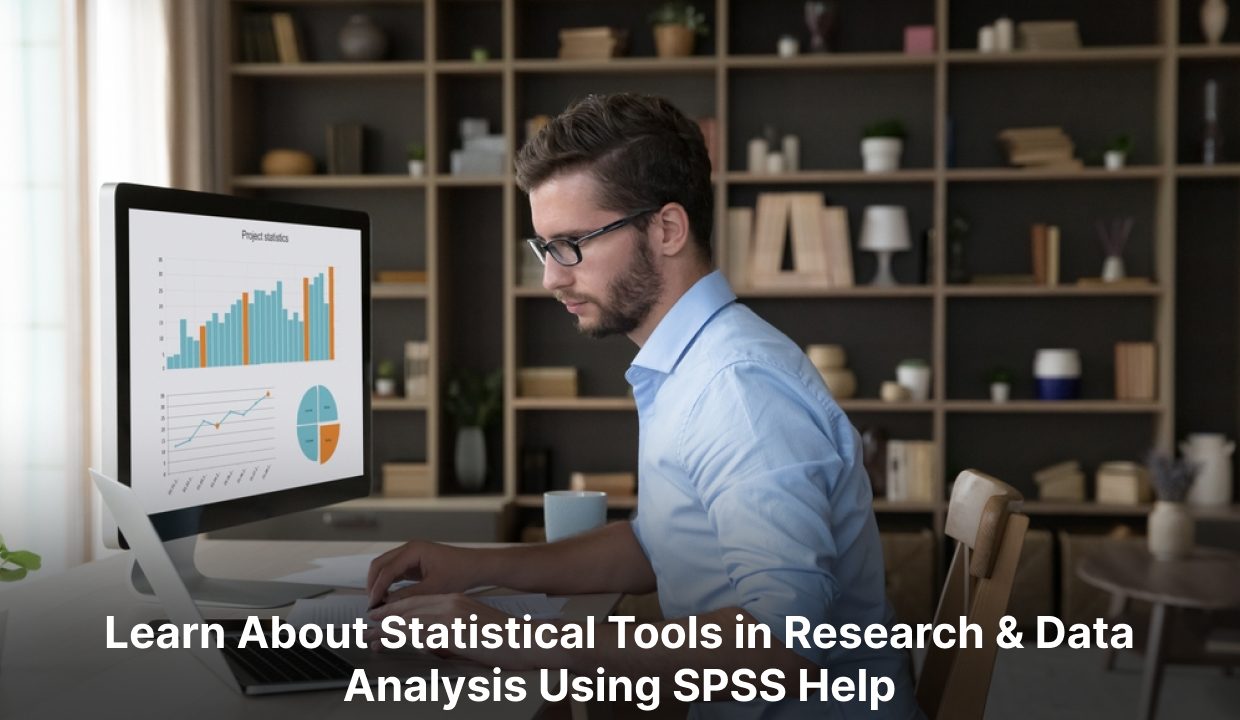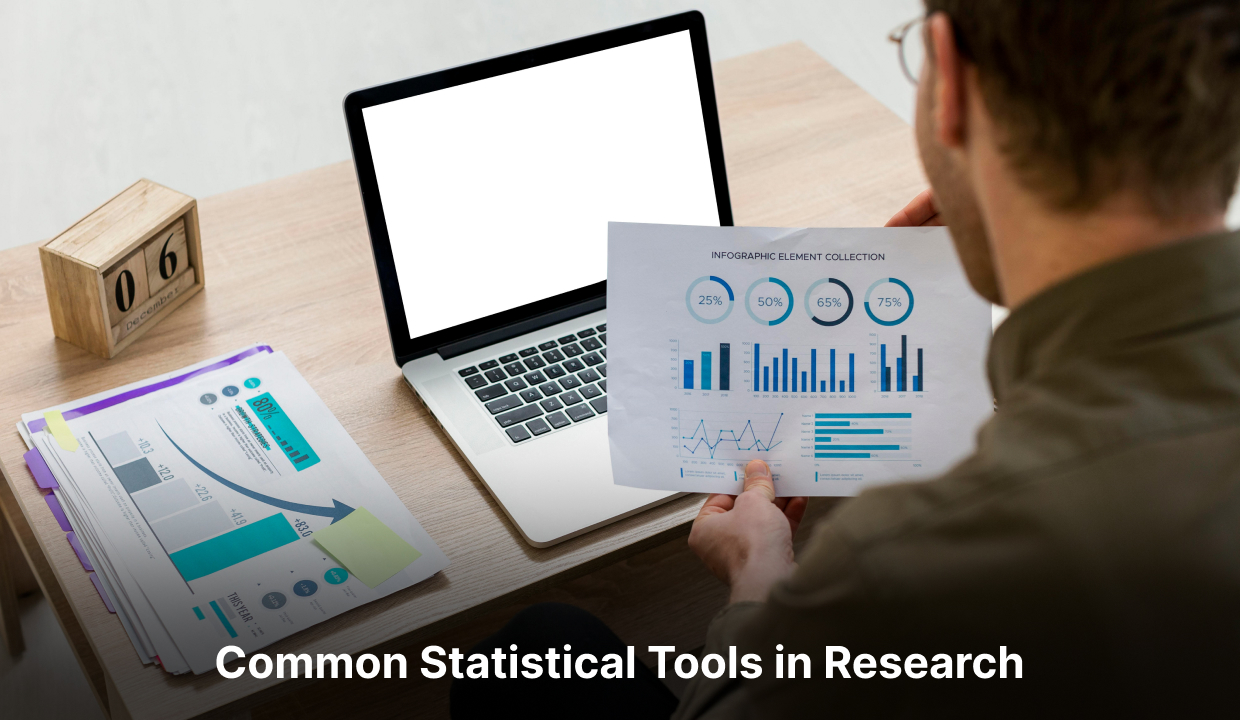# Power of Standard Statistical Tools in Research and Data Analysis## Introduction

Research and data analysis are vital across various domains, including scientific research and business decision-making. Standard statistical tools such as SPSS help are fundamental to these processes, enabling researchers to collect, organise, analyse, and interpret data. They provide valuable insights and support evidence-based conclusions. This blog will explore common statistical tools used in research and data analysis. Statistics is a scientific discipline that involves gathering, organising, analysing, and extrapolating data from samples to the entire population. Proper study design, sample selection, and appropriate statistical tests are crucial. A sound understanding of statistics is necessary for designing epidemiological research or clinical trials. Incorrect statistical approaches can lead to false findings and unethical practices.

## Exploring the Role of Quantitative Variables in Statistics

Quantitative variables play an essential role in statistics. They provide numerical information and allow mathematical operations and analysis. In statistics, quantitative variables are usually classified as continuous or discrete.
Continuous variables can take on any value within a specified range and are often measured on a scale. Height, weight, temperature, and time are a few examples of continuous variables. Continuous variables are usually analysed using descriptive statistics (such as mean, mean, and standard deviation) and predictive statistics such as correlation analysis and regression.
Discrete variables, on the other hand, can only take on specific values within a defined set. They are often numbered or classified. Discrete variables include:

1. The number of siblings a person has.
2. The number of people present in an assembly.
3. The outcome of a coin toss.

Discrete variables are usually analysed using frequency, bar charts, and probability distributions.
Quantitative variables are essential for conducting statistical analysis and drawing meaningful conclusions. Here are some critical aspects of their role in statistics:

1. Describing Data: Quantitative variables provide numerical information that allows for calculating summary statistics. Measures such as mean, median, mode, range, and standard deviation help describe the central tendency and variability of data.
2. Discovery of relationships: Quantitative variables enable the discovery of relationships between variables. Statistical techniques such as correlation analysis determine the strength and direction of associations between two or more quantitative variables.
3. Making Predictions: Quantitative variables are used in predictive modelling. Linear regression and time series analysis use historical data to forecast and predict future outcomes.
4. Hypothesis Testing: Quantitative variables are used to test hypotheses and determine the significance of relationships. Statistical tests, such as the t-test and analysis of variance (ANOVA), compare means or variances across different groups or conditions.
5. Decision Making: Quantitative variables provide the basis for decision-making and estimation. Statistical analyses help assess the effectiveness of interventions or treatments, evaluate the effect of variables on outcomes, and support evidence-based decision-making.

It is important to note that the appropriate statistical techniques and methods for analysing quantitative variables depend on the specific research question, the nature of the data, and the assumptions of the statistical model being used.

## Common statistical tools in research and data analysis

1. ### Descriptive Statistics

2. Descriptive statistics provide a summary of the main characteristics of a dataset. These include mean, median, mode, standard deviation, variance, range, and percentile. These statistics help researchers understand their data's central tendency, variability, and distribution.

• ### Inferential Statistics

Inferential statistics are used to make inferences or generalisations about a population based on a sample of data. They include hypothesis testing and estimation techniques such as confidence intervals. Inferential statistics help researchers draw conclusions and make predictions from their data.

• ### T-Test

T-Test is used to compare means between two groups or conditions. They determine whether there is a significant difference between the means of the two groups or if the difference is due to chance. Different types of t-tests exist, including independent samples t-tests, paired samples t-tests, and one-sample t-tests.

• ### Analysis of Variance (ANOVA)

ANOVA compares means among three or more groups or conditions. Determines whether there are significant differences between the means of multiple groups. ANOVA can be one-way (one independent variable) or factorial (multiple independent variables).

• ### Regression Analysis

Regression analysis is a statistical method to investigate the connection between a dependent variable and one or multiple independent variables. It helps researchers understand how changes in the independent variable affect the dependent variable. Simple linear regression, multiple regression, and logistic regression are common types of regression analysis.

• ### Chi-Square Test

Chi-Square Test assesses the relationship between two categorical variables. It determines whether the observed frequencies of the variable differ significantly from the expected frequencies. Chi-square tests are often used in contingency table analysis and goodness-of-fit tests.

• ### Correlation Analysis

Correlation analysis examines the relationship between two continuous variables. It measures the strength and direction of association using correlation coefficients such as Pearson's. Correlation analysis helps researchers understand the degree of linear relationship between variables.

• ### Factor Analysis

Factor analysis is used to detect patterns of correlations among a large set of variables. It identifies the underlying latent factors explaining the observed variables' relationship. Factor analysis is commonly used in psychometrics and the social sciences.

• ### Time series analysis

Time series analysis is applied to data collected over time to identify patterns, trends, and seasonality. It helps researchers predict future values and understand the data dynamics. Techniques used in time series analysis include the autoregressive integrated moving average (ARIMA) model and exponential smoothing.

• ### Cluster Analysis

Cluster analysis is used to classify objects or cases based on their similarity or dissimilarity. It helps to identify homogeneous subgroups within the dataset. Techniques such as K-means and hierarchical clustering are commonly used in cluster analysis.

##Conclusion

Standard statistical tools are vital for research and data analysis, enabling researchers to gain meaningful insights, make informed decisions, and draw valid conclusions. Descriptive statistics, inferential statistics, hypothesis testing, correlation analysis, and data visualisation are just a few examples of these tools. Effectively leveraging them allows researchers to navigate complex data, uncover relationships, and contribute to field advancements. A researcher must be well-versed in key statistical approaches to ensure a well-designed study that produces accurate and valid data. Incorrect statistical methods can lead to flawed findings, errors, and diminished research significance. Inadequate statistics can result in unethical behaviour. Therefore, a proper understanding of statistics and the appropriate application of statistical analysis is crucial. Good luck!